## Method

[la] STEAM-Lidar using Constant Acceleration Motion Prior [STEAM-L WNOJ]

Submitted on 17 Aug. 2018 23:45 by
Tim Tang (University of Toronto Institute for Aerospace Studies)

 Running time: 0.2 s Environment: 1 core @ 2.5 Ghz (C/C++)

 Method Description: Lidar odometry with STEAM, using a white-noise-on- jerk motion prior. Parameters: Specified in the upcoming paper Latex Bibtex: @article{tang2018white, title={A White-Noise-On-Jerk Motion Prior for Continuous-Time Trajectory Estimation on SE (3)}, author={Tang, Tim Y and Yoon, David J and Barfoot, Timothy D}, journal={arXiv preprint arXiv:1809.06518}, year={2018} }

## Detailed Results

From all test sequences (sequences 11-21), our benchmark computes translational and rotational errors for all possible subsequences of length (5,10,50,100,150,...,400) meters. Our evaluation ranks methods according to the average of those values, where errors are measured in percent (for translation) and in degrees per meter (for rotation). Details for different trajectory lengths and driving speeds can be found in the plots underneath. Furthermore, the first 5 test trajectories and error plots are shown below.

## Test Set Average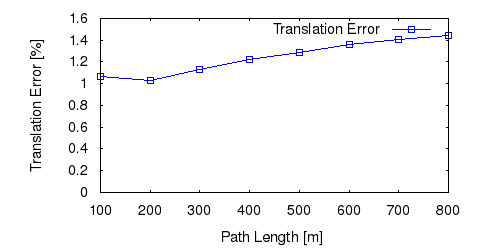This figure as: png eps pdf txt gnuplot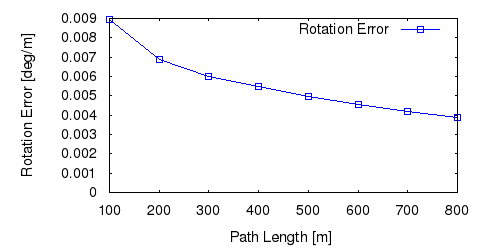This figure as: png eps pdf txt gnuplotThis figure as: png eps pdf txt gnuplot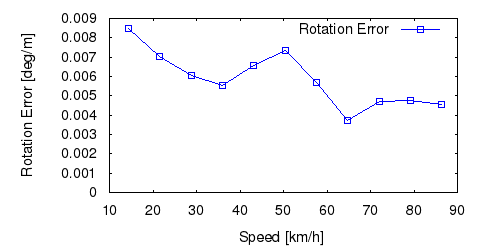This figure as: png eps pdf txt gnuplot

## Sequence 11This figure as: png eps pdf txt gnuplot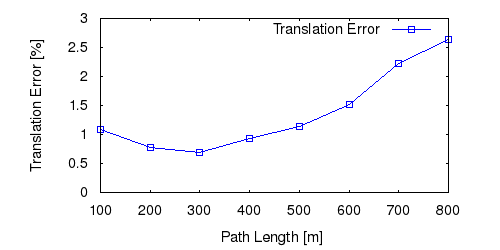This figure as: png eps pdf txt gnuplot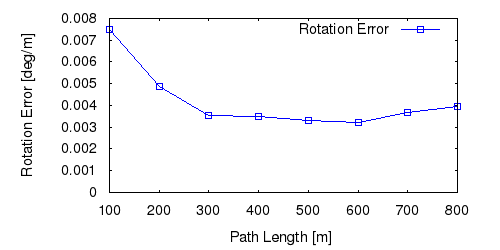This figure as: png eps pdf txt gnuplot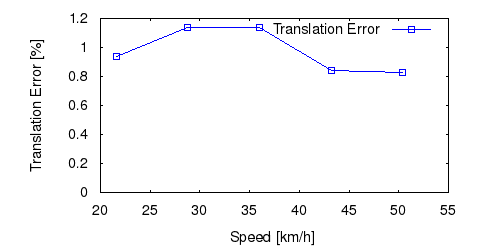This figure as: png eps pdf txt gnuplot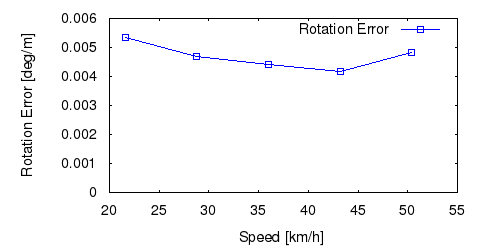This figure as: png eps pdf txt gnuplot

## Sequence 12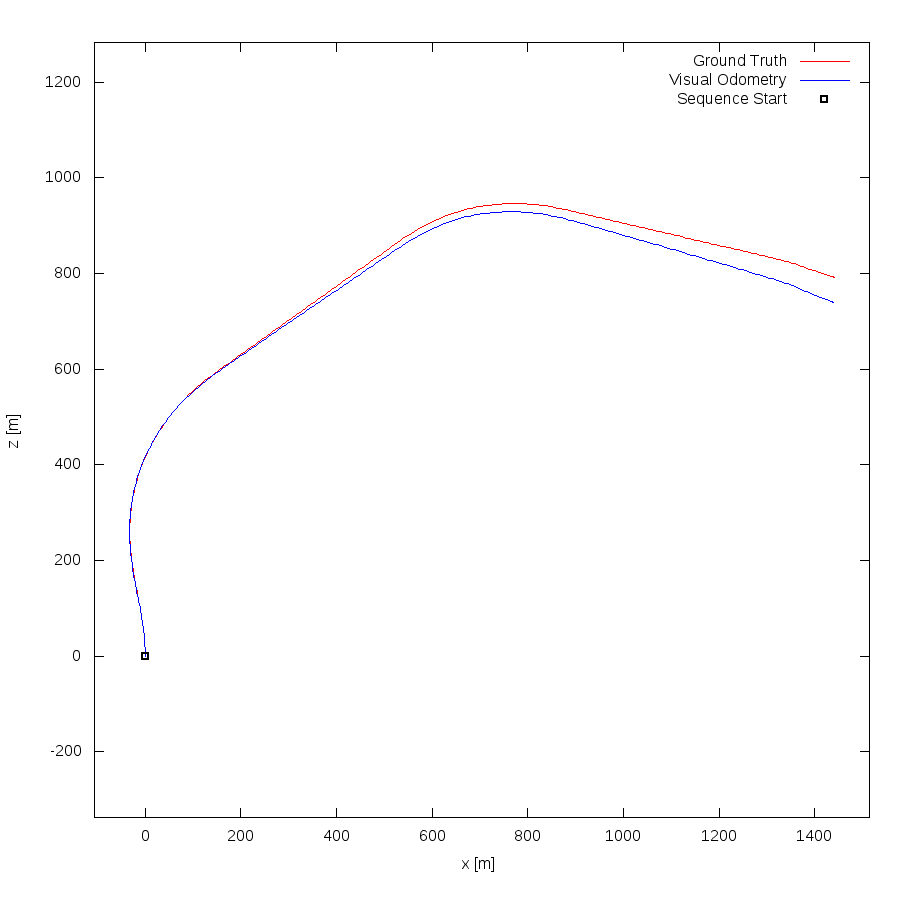This figure as: png eps pdf txt gnuplot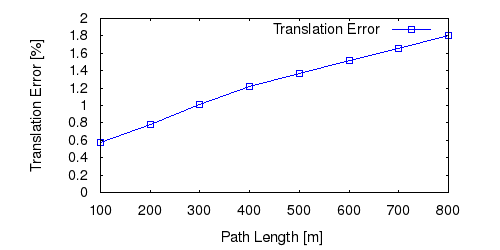This figure as: png eps pdf txt gnuplot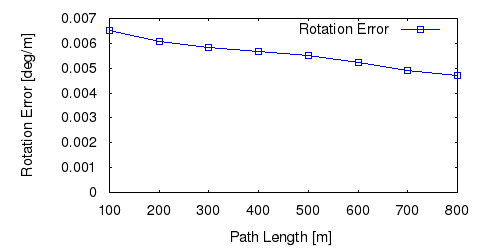This figure as: png eps pdf txt gnuplotThis figure as: png eps pdf txt gnuplotThis figure as: png eps pdf txt gnuplot

## Sequence 13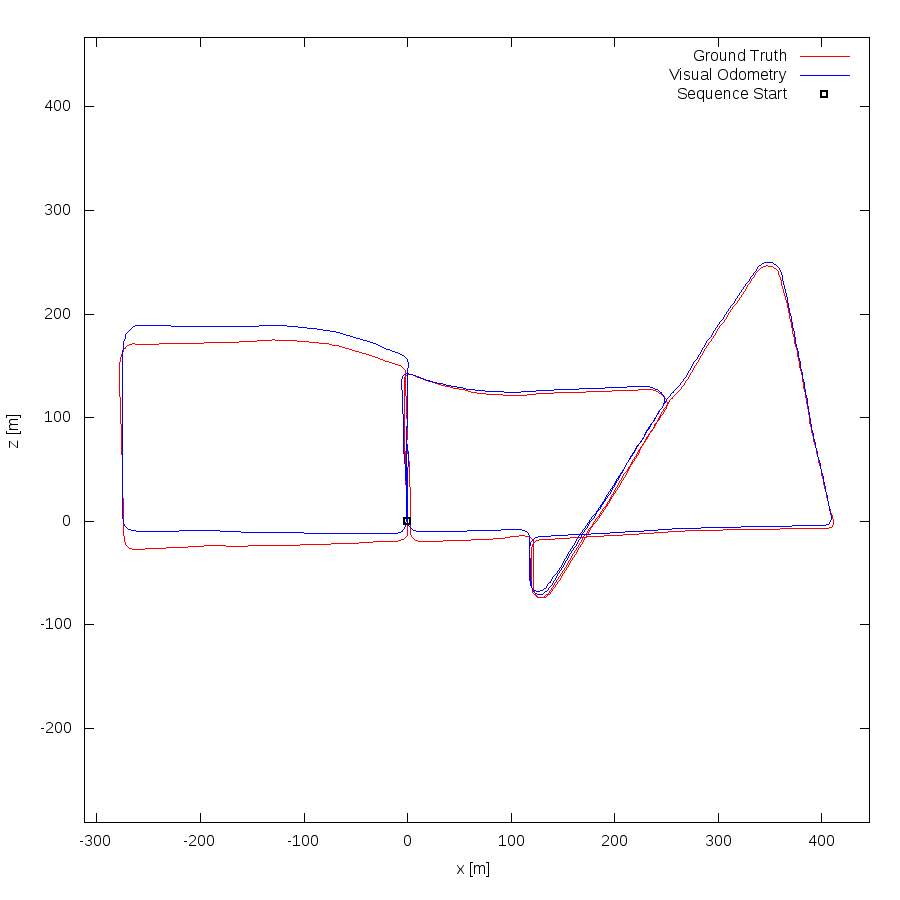This figure as: png eps pdf txt gnuplotThis figure as: png eps pdf txt gnuplot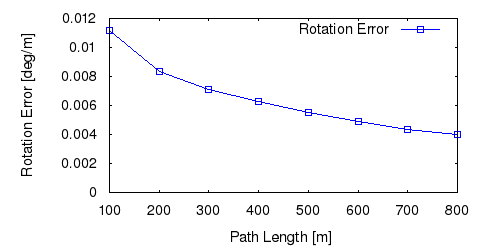This figure as: png eps pdf txt gnuplot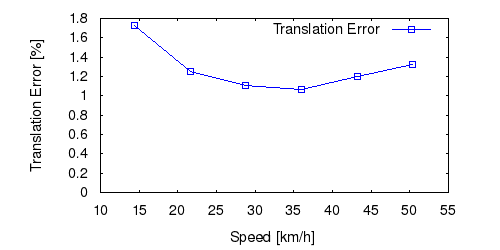This figure as: png eps pdf txt gnuplot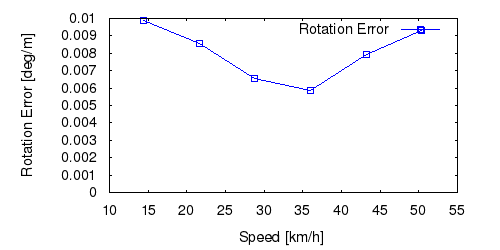This figure as: png eps pdf txt gnuplot

## Sequence 14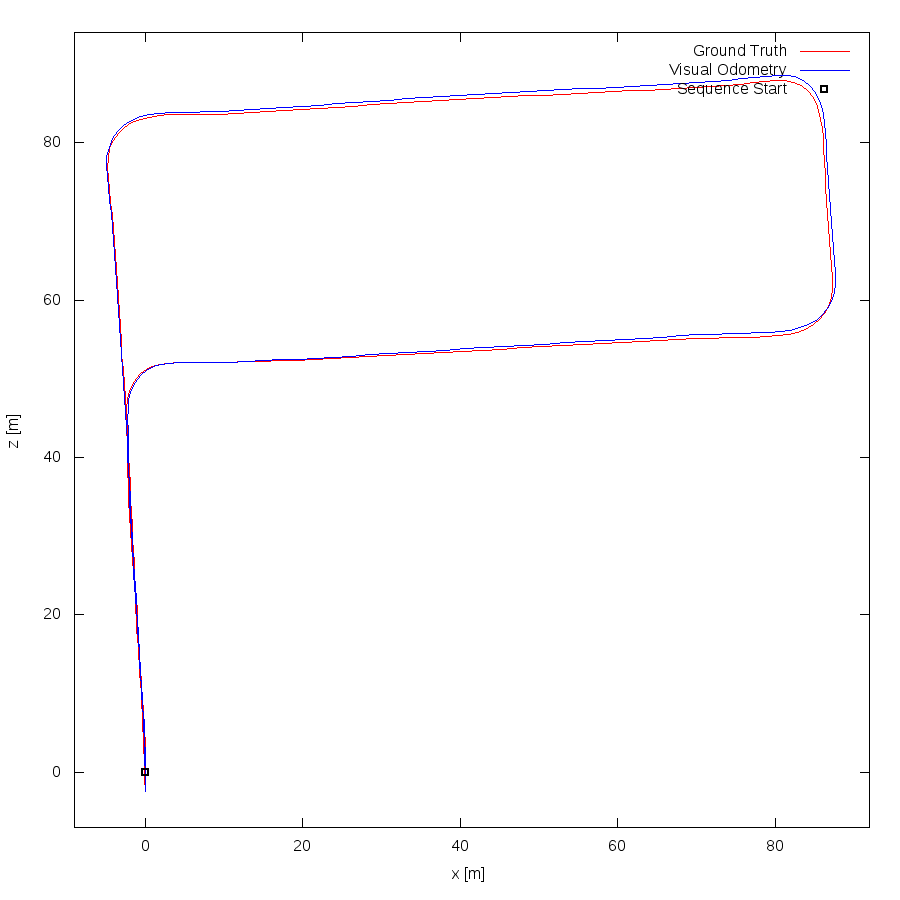This figure as: png eps pdf txt gnuplot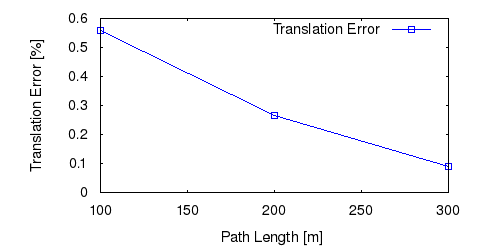This figure as: png eps pdf txt gnuplot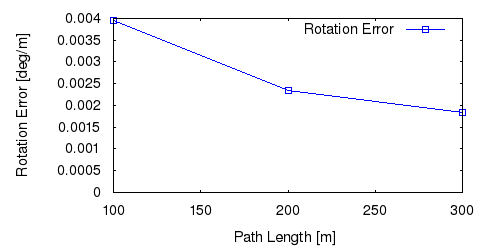This figure as: png eps pdf txt gnuplot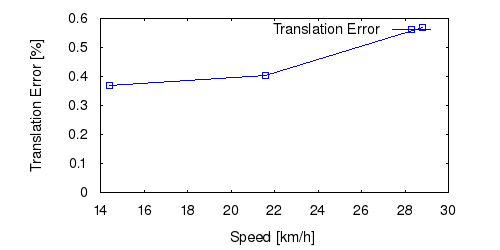This figure as: png eps pdf txt gnuplot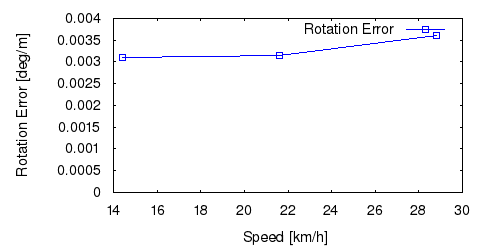This figure as: png eps pdf txt gnuplot

## Sequence 15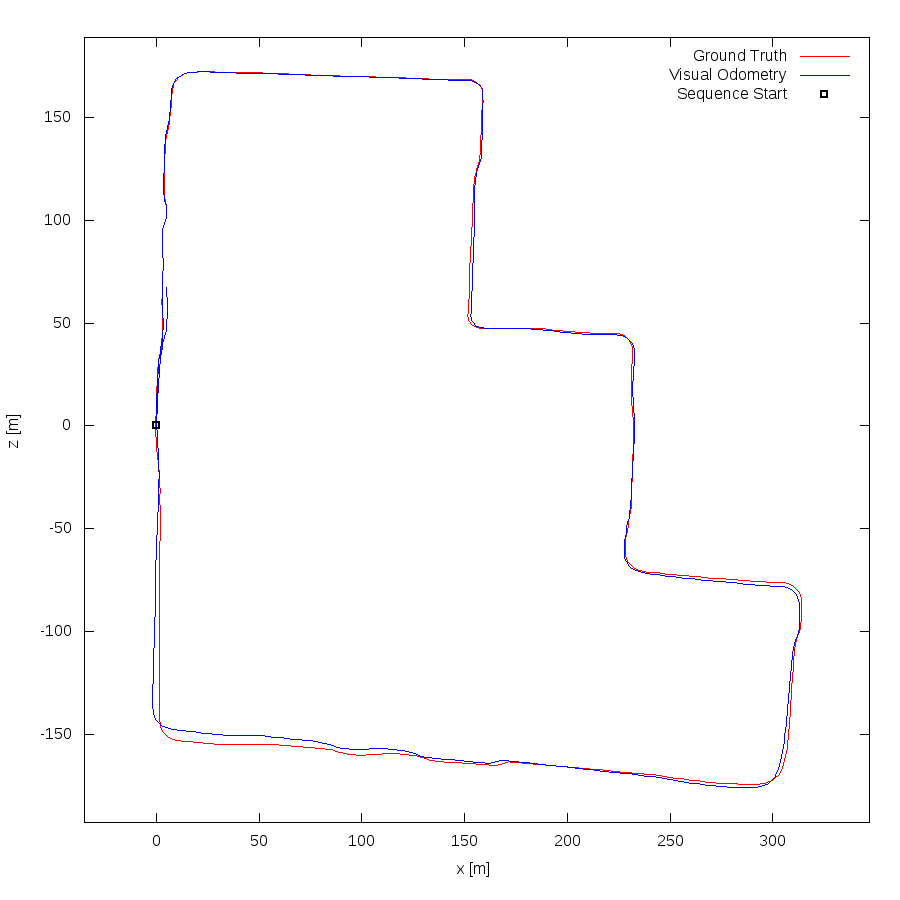This figure as: png eps pdf txt gnuplot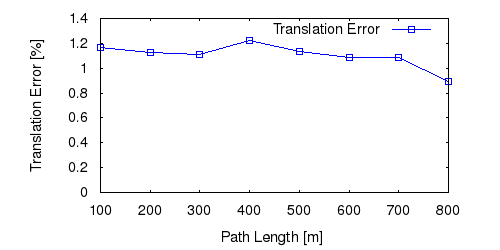This figure as: png eps pdf txt gnuplot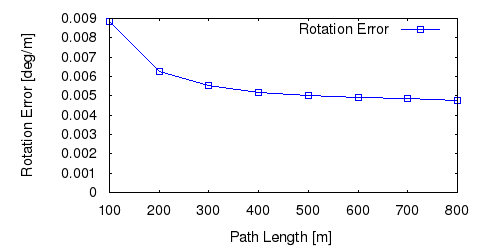This figure as: png eps pdf txt gnuplot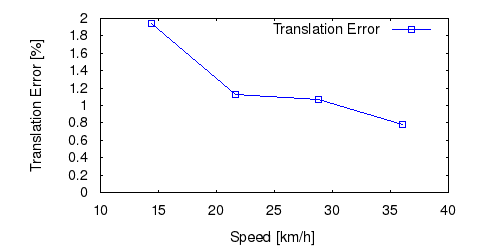This figure as: png eps pdf txt gnuplot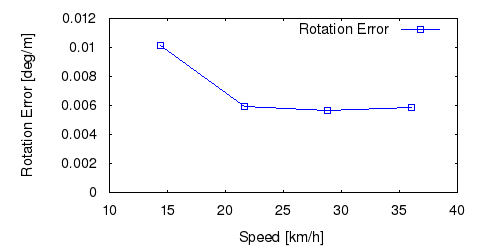This figure as: png eps pdf txt gnuplot